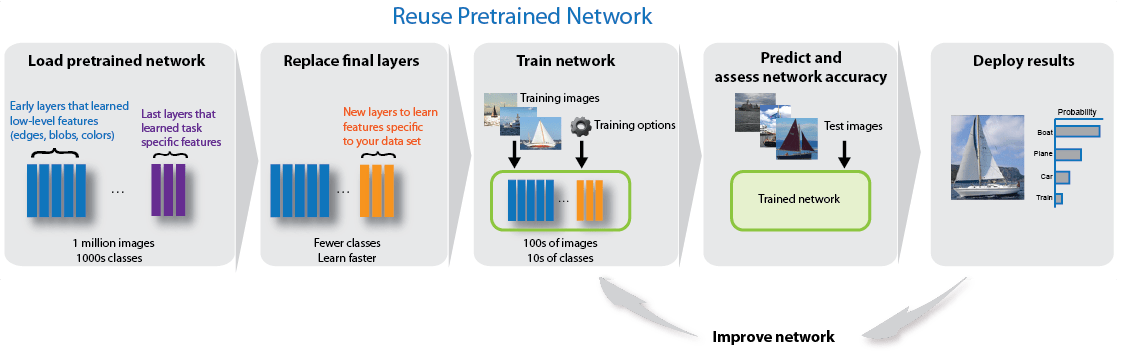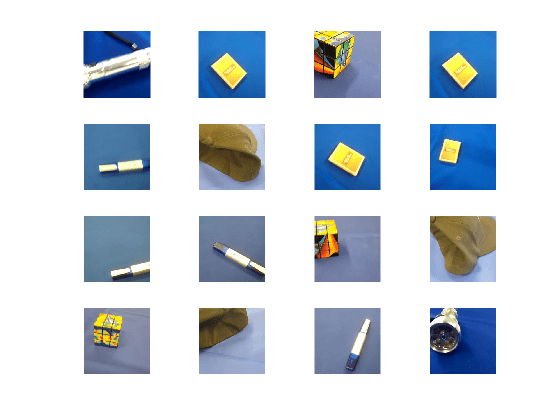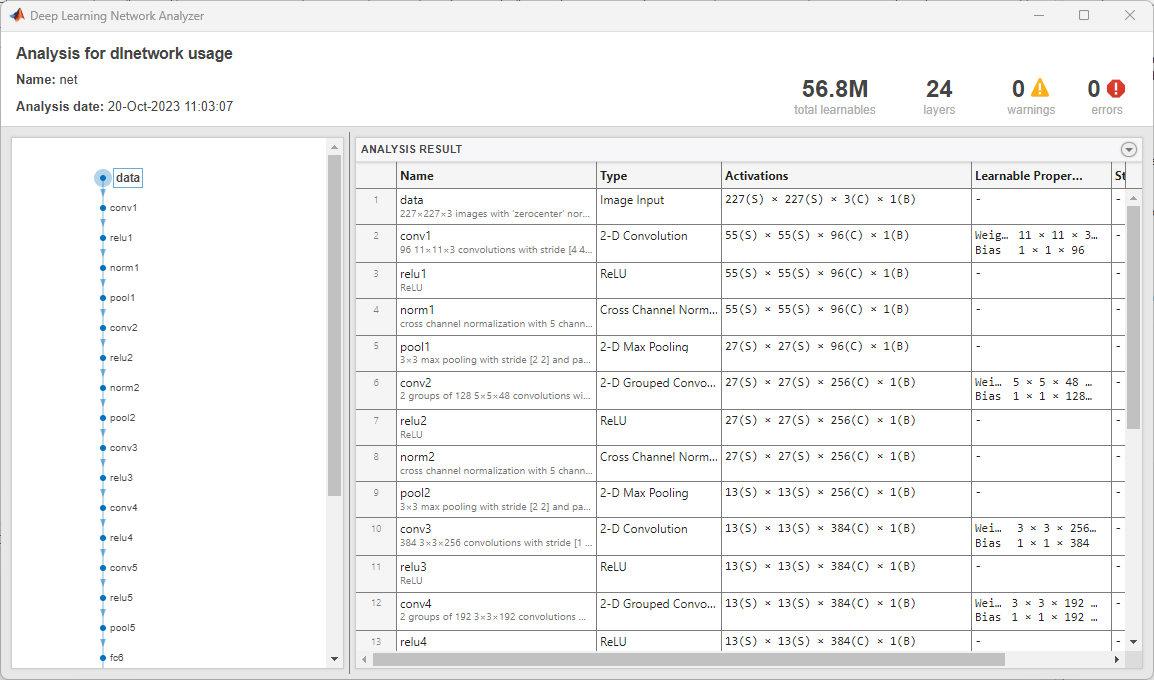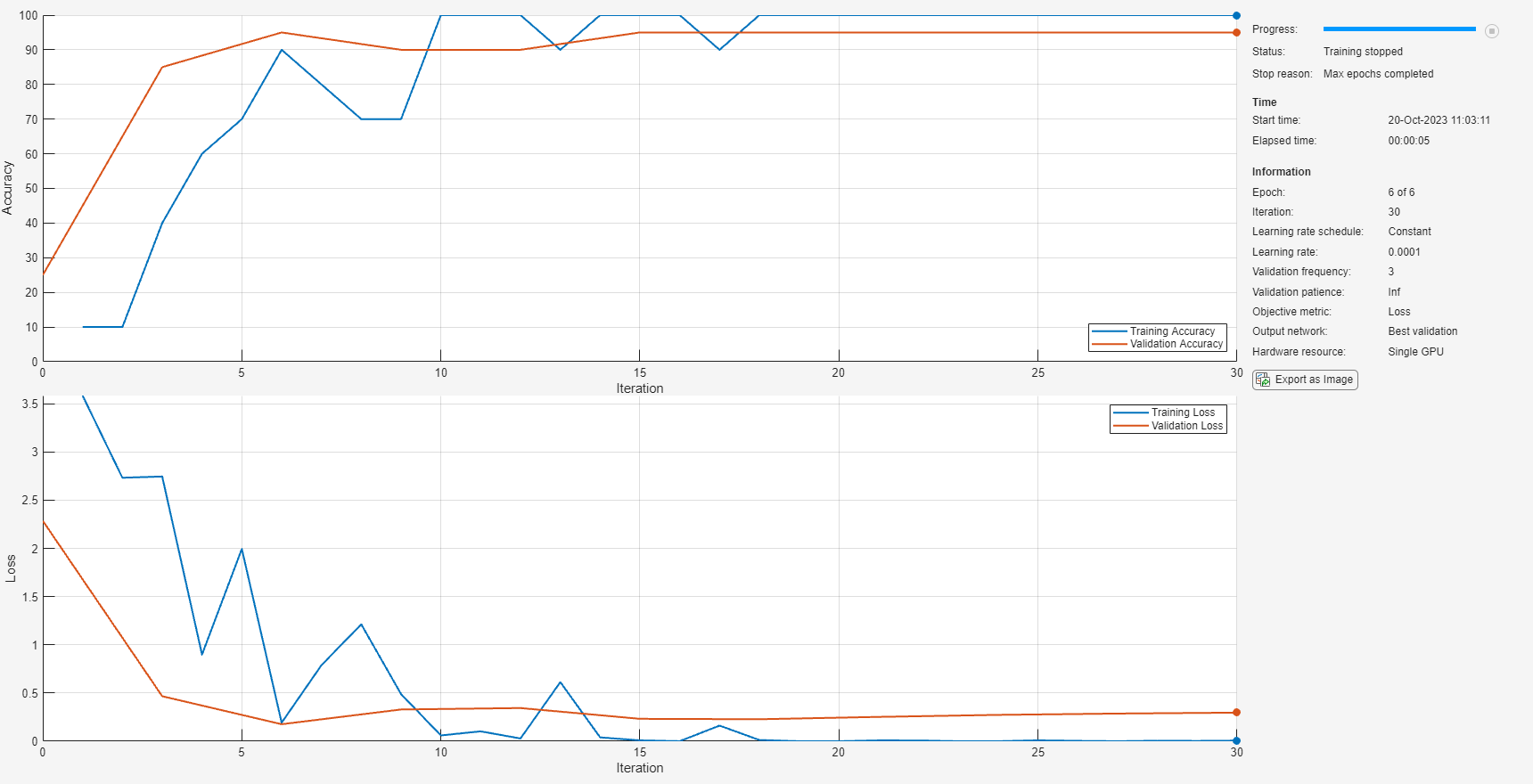# 使用 AlexNet 进行迁移学习

AlexNet 已基于超过一百万个图像进行训练，可以将图像分为 1000 个对象类别（例如键盘、咖啡杯、铅笔和多种动物）。该网络已基于大量图像学习了丰富的特征表示。网络以图像作为输入，然后输出图像中对象的标签以及每个对象类别的概率。### 加载数据

```unzip('MerchData.zip'); imds = imageDatastore('MerchData', ... 'IncludeSubfolders',true, ... 'LabelSource','foldernames');```

`[imdsTrain,imdsValidation] = splitEachLabel(imds,0.7,'randomized');`

```numTrainImages = numel(imdsTrain.Labels); idx = randperm(numTrainImages,16); figure for i = 1:16 subplot(4,4,i) I = readimage(imdsTrain,idx(i)); imshow(I) end```### 加载预训练网络

`net = alexnet;`

`analyzeNetwork(net)``inputSize = net.Layers(1).InputSize`
```inputSize = 1×3 227 227 3 ```

### 替换最终层

`layersTransfer = net.Layers(1:end-3);`

`numClasses = numel(categories(imdsTrain.Labels))`
```numClasses = 5 ```
```layers = [ layersTransfer fullyConnectedLayer(numClasses,'WeightLearnRateFactor',20,'BiasLearnRateFactor',20) softmaxLayer classificationLayer];```

### 训练网络

```pixelRange = [-30 30]; imageAugmenter = imageDataAugmenter( ... 'RandXReflection',true, ... 'RandXTranslation',pixelRange, ... 'RandYTranslation',pixelRange); augimdsTrain = augmentedImageDatastore(inputSize(1:2),imdsTrain, ... 'DataAugmentation',imageAugmenter);```

`augimdsValidation = augmentedImageDatastore(inputSize(1:2),imdsValidation);`

```options = trainingOptions('sgdm', ... 'MiniBatchSize',10, ... 'MaxEpochs',6, ... 'InitialLearnRate',1e-4, ... 'Shuffle','every-epoch', ... 'ValidationData',augimdsValidation, ... 'ValidationFrequency',3, ... 'Verbose',false, ... 'Plots','training-progress');```

`netTransfer = trainNetwork(augimdsTrain,layers,options);`### 对验证图像进行分类

`[YPred,scores] = classify(netTransfer,augimdsValidation);`

```idx = randperm(numel(imdsValidation.Files),4); figure for i = 1:4 subplot(2,2,i) I = readimage(imdsValidation,idx(i)); imshow(I) label = YPred(idx(i)); title(string(label)); end``````YValidation = imdsValidation.Labels; accuracy = mean(YPred == YValidation)```
```accuracy = 1 ```

 Krizhevsky, Alex, Ilya Sutskever, and Geoffrey E. Hinton. "ImageNet Classification with Deep Convolutional Neural Networks." Advances in neural information processing systems. 2012.

 BVLC AlexNet Model. https://github.com/BVLC/caffe/tree/master/models/bvlc_alexnet